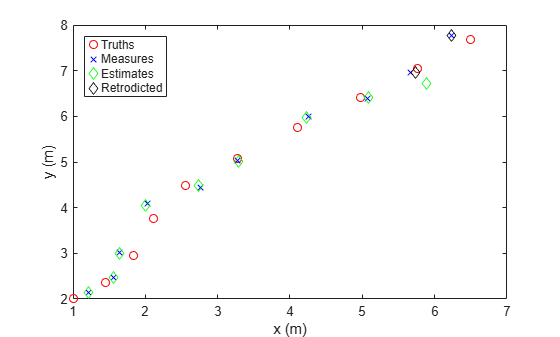retrodict

Retrodict filter to previous time step

Description

The retrodict function performs retrodiction, predicting the state estimate and covariance backward to the time at which an out-of-sequence measurement (OOSM) was taken. To use this function, specify the MaxNumOOSMSteps property of the filter as a positive integer. After using this function, use the retroCorrect function to update the current state estimates using the OOSM.

example

[retroState,retroCov] = retrodict(filter,dt) retrodicts the filter by time dt, and returns the retrodicted state and state covariance. The function also changes values of the State and StateCovariance properties of the filter object to retroState and retroCov, respectively.

[___,retrodictStatus] = retrodict(___) also returns the status of the retrodiction retrodictStatus as true for success and false for failure. The retrodiction process can fail if the length of the state history stored in the filter (specified by the MaxNumOOSMSteps property of the filter) does not cover the request time specified by the dt input.

Examples

collapse all

Generate a truth trajectory using the 3-D constant velocity model.

rng(2021) % For repeatable results
initialState = [1; 0.4; 2; 0.3; 1; -0.2]; % [x; vx; y; vy; z; vz]
dt = 1; % Time step
steps = 10;
sigmaQ = 0.2; % Standard deviation for process noise
states = NaN(6,steps);
states(:,1) = initialState;
for ii = 2:steps
w = sigmaQ*randn(3,1);
states(:,ii) = constvel(states(:,ii-1),w,dt);
end

Generate position measurements from the truths.

positionSelector = [1 0 0 0 0 0; 0 0 1 0 0 0; 0 0 0 0 1 0];
sigmaR = 0.2; % Standard deviation for measurement noise
positions = positionSelector*states;
measures = positions + sigmaR*randn(3,steps);

Show the truths and measurements in an x-y plot.

figure
plot(positions(1,:),positions(2,:),"ro","DisplayName","Truths");
hold on;
plot(measures(1,:),measures(2,:),"bx","DisplayName","Measures");
xlabel("x (m)")
ylabel("y (m)")
legend("Location","northwest")

Assume that, at the ninth step, the measurement is delayed and therefore unavailable.

delayedMeasure = measures(:,9);
measures(:,9) = NaN;

Construct an extended Kalman filter (EKF) based on the constant velocity model.

estimates = NaN(6,steps);
covariances = NaN(6,6,steps);

estimates(:,1) = positionSelector'*measures(:,1);
covariances(:,:,1) = 1*eye(6);
filter = trackingEKF(@constvel,@cvmeas,...
"State",estimates(:,1),...
"StateCovariance",covariances(:,:,1),...
"ProcessNoise",eye(6),...
"MeasurementNoise",sigmaR^2*eye(3),...
"MaxNumOOSMSteps",3);

Step through the EKF with the measurements.

for ii = 2:steps
predict(filter);
if ~any(isnan(measures(:,ii))) % Skip if unavailable
correct(filter,measures(:,ii));
end
estimates(:,ii) = filter.State;
covariances(:,:,ii) = filter.StateCovariance;
end

Show the estimated results.

plot(estimates(1,:),estimates(3,:),"gd","DisplayName","Estimates");

Retrodict to the ninth step, and correct the current estimates by using the out-of-sequence measurements at the ninth step.

[retroState,retroCov] = retrodict(filter,-1);
[retroCorrState,retroCorrCov] = retroCorrect(filter,delayedMeasure);

Plot the retrodicted state for the ninth step.

plot([retroState(1);retroCorrState(1)],...
[retroState(3),retroCorrState(3)],...
"kd","DisplayName","Retrodicted")You can use the determinant of the final state covariance to see the improvements made by retrodiction. A smaller covariance determinant indicates improved state estimates.

detWithoutRetrodiciton = det(covariances(:,:,end))
detWithoutRetrodiciton = 3.2694e-04
detWithRetrodiciton = det(retroCorrCov)
detWithRetrodiciton = 2.6063e-04

Input Arguments

collapse all

Tracking filter object, specified as a trackingKF or trackingEKF object.

Retrodiction time, in seconds, specified as a nonpositive integer. Specify retrodiction time as the time difference between the time at which the OOSM was taken and the current time.

Output Arguments

collapse all

Retrodicted state, returned as an M-by-1 real-valued vector, where M is the size of the filter state.

Retrodicted state covariance, returned as an M-by-M real-valued positive-definite matrix.

Retrodiction status, returned as true indicating success and false indicating failure.

collapse all

Retrodiction and Retro-Correction

Assume the current time step of the filter is k. At time k, the posteriori state and state covariance of the filter are x(k|k) and P(k|k), respectively. An out-of-sequence measurement (OOSM) taken at time β now arrives at time k. Find l such that β is a time step between these two consecutive time steps:

$k-l\le \beta

where l is a positive integer and l < k.

In the retrodiction step, the current state and state covariance at time k are predicted back to the time of the OOSM. You can obtain the retrodicted state by propagating the state transition function backward in time. For a linear state transition function, the retrodicted state is expressed as:

$x\left(\beta |k\right)=F\left(\beta ,k\right)x\left(k|k\right),$

where F(β,k) is the backward state transition matrix from time step k to time step β. The retrodicted covariance is obtained as:

$P\left(\beta |k\right)=F\left(\beta ,k\right)\left[P\left(k|k\right)+Q\left(k,\beta \right)-{P}_{xv}\left(\beta |k\right)-{P}_{xv}^{T}\left(\beta |k\right)\right]F{\left(\beta ,k\right)}^{T},$

where Q(k,β) is the covariance matrix for the process noise and,

${P}_{xv}=Q\left(k,\beta \right)-P\left(k|k-l\right){S}^{*}{\left(k\right)}^{-1}Q\left(k,\beta \right).$

Here, P(k|k-l) is the priori state covariance at time k, predicted from the covariance information at time k–l, and

${S}^{*}{\left(k\right)}^{-1}=P{\left(k|k-l\right)}^{-1}-P{\left(k|k-l\right)}^{-1}P\left(k|k\right)P{\left(k|k-l\right)}^{-1}.$

In the second step, retro-correction, the current state and state covariance are corrected using the OOSM. The corrected state is obtained as:

$x\left(k|\beta \right)=x\left(k|k\right)+W\left(k,\beta \right)\left[z\left(\beta \right)-z\left(\beta |k\right)\right],$

where z(β) is the OOSM at time β and W(k,β), the filter gain, is expressed as:

$W\left(k,\beta \right)={P}_{xz}\left(\beta |k\right){\left[H\left(\beta \right)P\left(\beta |k\right){H}^{T}\left(\beta \right)+R\left(\beta \right)\right]}^{-1}.$

You can obtain the equivalent measurement at time β based on the state estimate at the time k, z(β|k), as

$z\left(\beta |k\right)=H\left(\beta \right)x\left(\beta |k\right).$

In these expressions, R(β) is the measurement covariance matrix for the OOSM and:

${P}_{xz}\left(\beta |k\right)=\left[P\left(k|k\right)-{P}_{xv}\left(\beta |k\right)\right]F{\left(\beta ,k\right)}^{T}H{\left(\beta \right)}^{T},$

where H(β) is the measurement Jacobian matrix. The corrected covariance is obtained as:

$P\left(k|\beta \right)=P\left(k|k\right)-{P}_{xz}\left(\beta |k\right)S{\left(\beta \right)}^{-1}{P}_{xz}{\left(\beta |k\right)}^{T}.$

 Bar-Shalom, Y., Huimin Chen, and M. Mallick. “One-Step Solution for the Multistep out-of-Sequence-Measurement Problem in Tracking.” IEEE Transactions on Aerospace and Electronic Systems 40, no. 1 (January 2004): 27–37. https://doi.org/10.1109/TAES.2004.1292140.